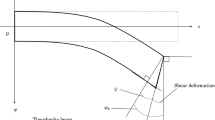# Continuity of the flow map for symmetric hyperbolic systems and its application to the Euler-Poisson system

## Abstract

We show the continuity of the flow map for quasilinear symmetric hyperbolic systems with general right-hand sides in different functional setting, including weighted Sobolev spaces Hs δ. An essential tool to achieve the continuity of the flow map is a new type of energy estimate, which we call a low regularity energy estimate. We then apply these results to the Euler-Poisson system which describes various systems of physical interest.

This is a preview of subscription content, log in via an institution to check access.

Price excludes VAT (USA)
Tax calculation will be finalised during checkout.

### Similar content being viewed by others### Pressure Function and Limit Theorems for Almost Anosov Flows

Henk Bruin, Dalia Terhesiu & Mike Todd

### SBV Regularity of Systems of Conservation Laws and Hamilton–Jacobi Equations

S. BianchiniNaofumi Mori

## References

1. H. Beirão da Veiga, Data dependence in the mathematical theory of compressible inviscid fluids, Arch. Rational Mech. Anal. 119 (1992), 109–127.

2. H. Bahouri, J. Chemin and R. Danchin, Fourier Analysis and Nonlinear Partial Differential Equations, Springer, Heidelberg-London-New York, 2011.

3. U. Brauer and L. Karp, Local existence of solutions of self gravitating relativistic perfect fluids, Comm. Math. Phys. 325 (2014), 105–141.

4. U. Brauer and L. Karp, Elliptic equations in weighted Besov spaces on asymptotically flat Riemannian manifolds, Manuscripta Math. 148 (2015), 59–97.

5. U. Brauer and L. Karp, Local existence of solutions to the Euler-Poisson system, including densities without compact support, J. Differential Equations 264 (2018), 755–785.

6. U. Brauer, An existence theorem for perturbed newtonian cosmological models, J. Math. Phys. 33 (1992), 1224–1233.

7. M. Cantor, Spaces of functions with asymptotic conditions onn, Indiana Univ. Math. J. 24 (1975), 897–902.

8. Y. Choquet-Bruhat and D. Christodoulou, Elliptic systems in Hs,δ spaces on manifolds which are euclidian at infinity, Acta Math. 146 (1981), 129–150.

9. D. Christodoulou, The Action Principle and Partial Differential Equations, Princeton University Press, Princeton, NJ, 2000.

10. A. E. Fischer and J. E. Marsden, The Einstein evolution equations as a first-order quasi-linear symmetric hyperbolic system, Comm. Math. Phys. 28 (1972), 1–38.

11. T. Kato, The Cauchy problem for quasi-linear symmetric hyperbolic systems, Arch. Ration. Mech. Anal. 58 (1975), 181–205.

12. T. Kato, Quasi-linear equations of evolution, with applications to partial differential equations, in Spectral Theory and Differential Equations, Springer, Berlin, 1975, pp. 25–70.

13. D. Kateb, On the boundedness of the mapping f → |f|µ, µ 1 on Besov spaces, Math. Nachr. 248/249 (2003), 110–128.

14. T. Kato and C. Y. Lai, Nonlinear evolution equations and the Euler flow, J. Funct. Anal. 56 (1984), 15–28.

15. T. Kato and G. Ponce, Commutator estimates and the Euler and Navier-Stokes equations, Comm. Pure Appl. Math. 41 (1988), 891–907.

16. J. M. Lee and T. H. Parker, The Yamabe problem, Bull. Amer. Math. Soc. (N.S.) 17 (1987), 37–91.

17. A. Majda, Compressible Fluid Flow and Systems of Conservation Laws in Several Space Variables, Springer, New York, 1984.

18. T. Makino, On a local existence theorem for the evolution equation of gaseous stars, in Patterns and Waves, North Holland, Amsterdam, 1986, pp. 459–479.

19. D. Maxwell, Rough solutions of the Einstein constraint equations, J. Reine Angew. Math. 590 (2006), 1–29.

20. L. Nirenberg and H. Walker, The null spaces of elliptic differential operators inn, J. Math. Anal. Appl. 42 (1973), 271–301.

21. J. Rauch, Hyperbolic Partial Differential Equations and Geometric Optics, American Mathematical Society, Providence, RI, 2012.

22. W. Rindler, Essential Relativity, Springer, Berlin-Heidelberg, 1978.

23. G. Rein and A. D. Rendall, Global existence ofclassical solutions to the Vlasov-Poisson system in a three-dimensional, cosmological setting, Arch. Rational Mech. Anal. 126 (1994), 183–201.

24. T. Runst and W. Sickel, Sobolev Spaces of Fractional Order, Nemytskij Operators, and Nonlinear Partial Differential Equations, Walter de Gruyter, Berlin, 1996.

25. J. Speck, Well-posedness for the Euler-Nordstrom system with cosmological constant, J. Hyperbolic Differ. Equ. 6 (2009), 313–358.

26. E. Stein, Singular Integrals and Differentiability Properties of Functions, Princeton University Press, Princeton, NJ, 1970.

27. T. Tao, Nonlinear Dispersive Equations, American Mathematical Society, Providence, RI, 2006.

28. M. Taylor, Pseudodifferential Operators and Nonlinear PDE, Birkhauser, Boston, MA, 1991.

29. M. Taylor, Partial Differential Equations. vol III. Nonlinear Equations, Springer, New York, 1997.

30. R. Temam, Navier-Stokes Equations, North-Holland, Amsterdam, 1979.

31. H. Triebel, Spaces of Kudrjavcev type I. Interpolation, embedding, and structure, J. Math. Anal. Appl. 56 (1976), 253–277.

32. N. Tzvetkov, Ill-posedness issues for nonlinear dispersive equations, in Lectures on Nonlinear Dispersive Equations, Gakkbōtosho, Tokyo, 2006, pp. 63–103.

## Author information

Authors

### Corresponding author

Correspondence to Lavi Karp.